Main Content

# Specify Axis Limits

You can control where data appears in the axes by setting the x-axis, y-axis, and z-axis limits. You also can change where the x-axis and y-axis lines appear (2-D plots only) or reverse the direction of increasing values along each axis.

### Change Axis Limits

Create a line plot. Specify the axis limits using the `xlim` and `ylim` functions. For 3-D plots, use the `zlim` function. Pass the functions a two-element vector of the form `[min max]`.

```x = linspace(-10,10,200); y = sin(4*x)./exp(x); plot(x,y) xlim([0 10]) ylim([-0.4 0.8])```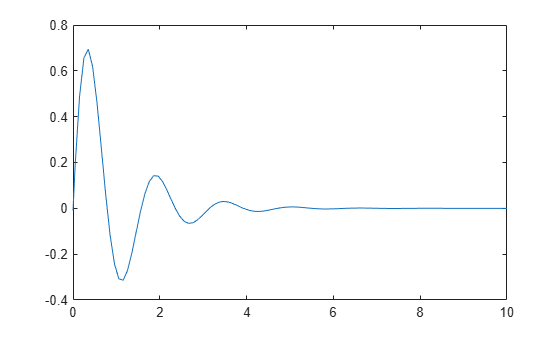### Use Semiautomatic Axis Limits

Set the maximum x-axis limit to 0 and the minimum y-axis limit to -1. Let MATLAB choose the other limits. For an automatically calculated minimum or maximum limit, use `-inf` or `inf`, respectively.

```[X,Y,Z] = peaks; surf(X,Y,Z) xlabel('x-axis') ylabel('y-axis') xlim([-inf 0]) ylim([-1 inf])```### Revert Back to Default Limits

Create a mesh plot and change the axis limits. Then revert back to the default limits.

```[X,Y,Z] = peaks; mesh(X,Y,Z) xlim([-2 2]) ylim([-2 2]) zlim([-5 5])```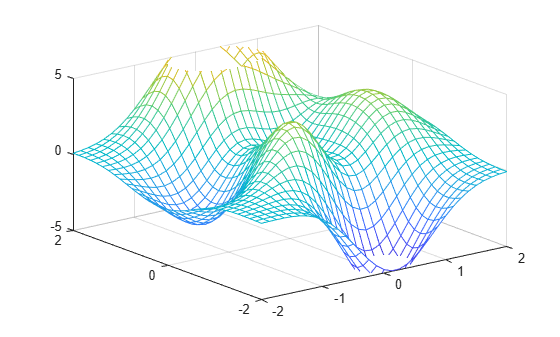```xlim auto ylim auto zlim auto```### Reverse Axis Direction

Control the direction of increasing values along the x-axis and y-axis by setting the `XDir` and `YDir` properties of the `Axes` object. Set these properties to either `'reverse'` or `'normal'` (the default). Use the `gca` command to access the `Axes` object.

```stem(1:10) ax = gca; ax.XDir = 'reverse'; ax.YDir = 'reverse';```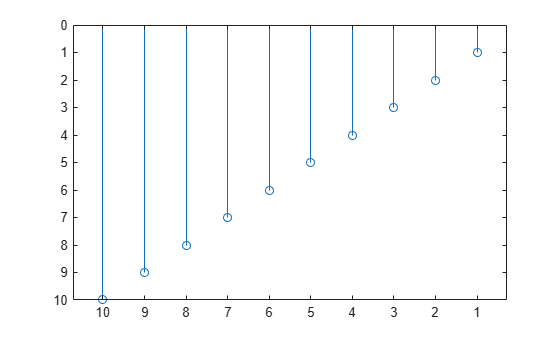### Display Axis Lines through Origin

By default, the x-axis and y-axis appear along the outer bounds of the axes. Change the location of the axis lines so that they cross at the origin point `(0,0)` by setting the `XAxisLocation` and `YAxisLocation` properties of the `Axes` object. Set `XAxisLocation` to either `'top'`, `'bottom'`, or `'origin'`. Set `YAxisLocation` to either `'left'`, `'right'`, or `'origin'`. These properties only apply to axes in a 2-D view.

```x = linspace(-5,5); y = sin(x); plot(x,y) ax = gca; ax.XAxisLocation = 'origin'; ax.YAxisLocation = 'origin';```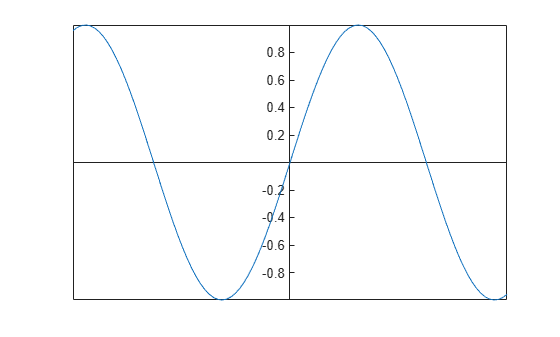Remove the axes box outline.

`box off`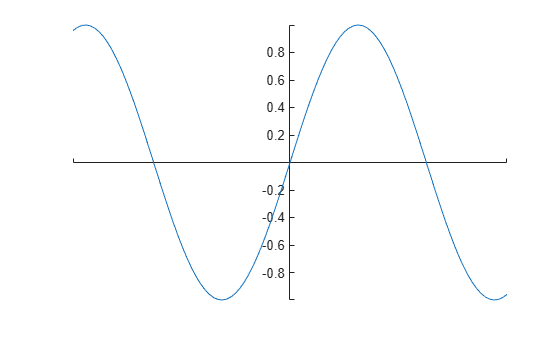Download ebook